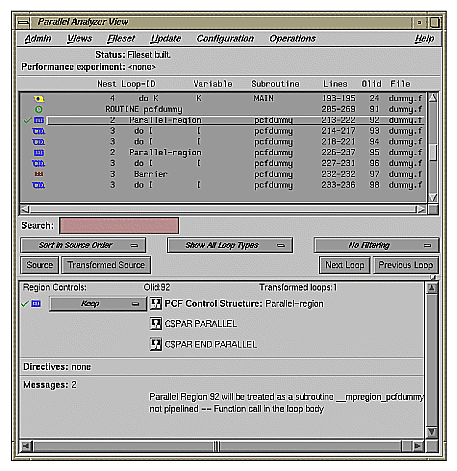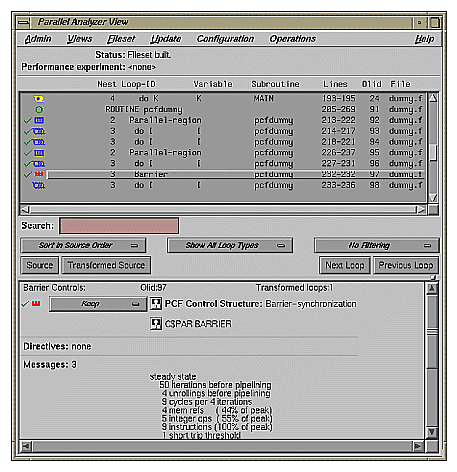## Appendix A. Examining Loops Containing PCF Directives

The content of this appendix is similar to that of “Examples Using OpenMP Directives” in Chapter 2, except it uses the older PCF (Parallel Computing Forum) directives instead of OpenMP directives.

## Setting Up the dummy.f Sample Session

To use this sample session, note the following:

• /usr/demos/ProMP is the PCF demonstration directory

• ProMP.sw.demos must be installed

The sample session discussed in this chapter uses the following source files in the directory /usr/demos/ProMP/tutorial :

• dummy.f_orig

• pcf.f_orig

• reshape.f_orig

• dist.f_orig

The source files contain many DO loops, each of which exemplifies an aspect of the parallelization process.

The directory /usr/demos/ProMP/tutorial also includes Makefile to compile the source files.

## Compiling the Sample Code

Prepare for the session by opening a shell window and entering the following:

 ```% cd /usr/demos/ProMP/tutorial % make ```

This creates the following files:

• dummy.f: a copy of the demonstration program created by combining the *.f_orig files, which you can view with the Parallel Analyzer View or a text editor, and print

• dummy.m: a transformed source file, which you can view with the Parallel Analyzer View, and print

• dummy.l: a listing file

• dummy.anl: an analysis file used by the Parallel Analyzer View

## Starting the Parallel Analyzer View

Once you have created the appropriate files with the compiler, start the session by entering the following command, which opens the main window of the Parallel Analyzer View loaded with the sample file data:

 `% cvpav -f dummy.f `

Open the Source View window by clicking the Source button after the Parallel Analyzer View main window opens.

## Examples Using PCF Directives

This section discusses the subroutine pcfdummy() , which contains four parallel regions and a single-process section that illustrate the use of PCF directives:

To go to the first explicitly parallelized loop in pcfdummy() , scroll down the loop list to Olid 92.

Select this loop by double-clicking the highlighted line in the loop list.

### Explicitly Parallelized Loops: C\$PAR PDO

The first construct in subroutine pcfdummy() is a parallel region, Olid 92, containing two loops that are explicitly parallelized with C\$PAR PDO statements. (See Figure A-1.) With this construct, the second loop can start before all iterations of the first complete.

Example A-1. Explicitly Parallelized Loop Using C\$PAR PDO

 ```C\$PAR PARALLEL SHARED(A,B) LOCAL(I) C\$PAR PDO dynamic blocked(10-2*2) DO 6001 I=-100,100 A(I) = I 6001 CONTINUE C\$PAR PDO static DO 6002 I=-100,100 B(I) = 3 * A(I) 6002 CONTINUE C\$PAR END PARALLEL```

Notice in the loop information display that the parallel region has controls for the region as a whole. The Keep option button and the highlight buttons function the same way they do in the Loop Parallelization Controls.

Click Next Loop twice to step through the two loops. You can see in the Source View that both loops contain a C\$PAR PDO directive.

Click Next Loop to step to the second parallel region.

Figure A-1. Explicitly Parallelized Loops Using C\$PAR PDO### Loops With Barriers: C\$PAR BARRIER

The second parallel region, Olid 95, contains a pair of loops identical to the previous example, but with a barrier between them. Because of the barrier, all iterations of the first C\$PAR PDO must complete before any iteration of the second loop can begin.

Example A-2. Loops Using C\$PAR BARRIER

 ```C\$PAR PARALLEL SHARED(A,B) LOCAL(I) C\$PAR PDO interleave blocked(10-2*2) DO 6003 I=-100,100 A(I) = I 6003 CONTINUE C\$PAR END PDO NOWAIT C\$PAR barrier C\$PAR PDO static DO 6004 I=-100,100 B(I) = 3 * A(I) 6004 CONTINUE C\$PAR END PARALLEL```

Click Next Loop twice to view the barrier region. (See Figure A-2.)

Click Next Loop twice to go to the third parallel region.

Figure A-2. Loops Using C\$PAR BARRIER Synchronization### Critical Sections: C\$PAR CRITICAL SECTION

Click Next Loop to view the first of the two loops in the third parallel region, Olid 100. This loop contains a critical section.

Example A-3. Critical Section Using C\$PAR CRITICAL SECTION

 ```C\$PAR PDO DO 6005 I=1,100 C\$PAR CRITICAL SECTION (S3) S1 = S1 + I C\$PAR END CRITICAL SECTION 6005 CONTINUE```

Click Next Loop to view the critical section.

The critical section uses a named locking variable (S3 ) to prevent simultaneous updates of S1 from multiple threads. This is a standard construct for performing a reduction.

Move to the next loop by clicking Next Loop .

### Single-Process Sections: C\$PAR SINGLE PROCESS

Loop Olid 102 has a single-process section, which ensures that only one thread can execute the statement in the section. Highlighting in the Source View shows the begin and end directives.

Example A-4. Single-Process Section Using C\$PAR SINGLE PROCESS

 ``` DO 6006 I=1,100 C\$PAR SINGLE PROCESS S2 = S2 + I C\$PAR END SINGLE PROCESS 6006 CONTINUE```

Click Next Loop to view information about the single-process section.

Move to the final parallel region in pcfdummy() by clicking Next Loop.

### Parallel Sections: C\$PAR PSECTIONS

The fourth and final parallel region of pcfdummy() , Olid 104, provides an example of parallel sections. In this case, there are three parallel subsections, each of which calls a function. Each function is called exactly once, by a single thread. If there are three or more threads in the program, each function may be called from a different thread. The compiler treats this directive as a single-process directive, which guarantees correct semantics.

Example A-5. Parallel Section Using C\$PAR PSECTIONS

 ```C\$PAR PARALLEL shared(a,c) local(i,j) C\$PAR PSECTIONS call boo C\$PAR SECTION call bar C\$PAR SECTION call baz C\$PAR END PSECTIONS C\$PAR END PARALLEL```

Click Next Loop to view the parallel section.

## Exiting From the dummy.f Sample Session

This completes the PCF sample session.

Close the Source View window by choosing its File -> Close option.

Quit the Parallel Analyzer View by choosing Admin -> Exit.

To clean up the directory, enter the following in your shell window to remove all of the generated files:

 `% make clean `